Definitions of Square Dance Calls and Concepts
Turn The Key [C3B]

Index -->  Plus  |  A1  |  A2  |  C1  |  C2  |  C3A  |  C3B  |  C4  |  NOL  |
Definitions (Text Only) -->  Plus  |  A1  |  A2  |  C1  |  C2  |  C3A  |  C3B  |  C4  |  NOL  |
 Find call:

 \$B8@8l(B\$BE,@Z\$J(B formation \$B\$+\$i(B.

2. Counter Rotate 1/4;
3. Hinge.

\$BMM!9\$J(B formation \$B\$G=*\$o\$j\$^\$9(B. Turn The Key \$B\$O(B 3 \$B%Q!<%H\$N%3!<%k\$G\$9(B.

3 & 1 Lines \$B\$+\$i(B: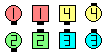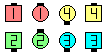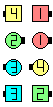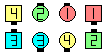Turn The Key\$B\$NA0(B Trade\$B\$N8e(B Counter Rotate 1/4\$B\$N8e(B Hinge\$B\$N8e(B (\$B=*\$o\$j(B)
1/4 Tag \$B\$+\$i(B: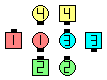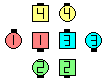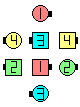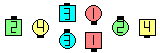Turn The Key\$B\$NA0(B Trade\$B\$N8e(B Counter Rotate 1/4\$B\$N8e(B Hinge\$B\$N8e(B (\$B=*\$o\$j(B)

\$BCm
• The Counter Rotate 1/4 \$B\$O(B, Concentric ('\$BBg\$-\$\$J}\$N(B') Counter Rotate 1/4 \$B\$G\$9(B.
• Turn The Key \$B\$r@.8y\$5\$;\$k\$K\$O(B, \$B%A!<%`%o!<%/\$,I,MW\$G\$9(B. \$B3F!9\$N9=@.MWAG\$N%3!<%k\$r@53N\$K9T\$\$(B, \$B5^\$,\$J\$\$\$3\$H\$,Bg@Z\$G\$9(B.
• Split Turn The Key [C3B] : Split \$B\$G(B Turn The Key \$B\$r\$7\$^\$9(B (\$B\$9\$J\$o\$A(B, Trade; Split Counter Rotate 1/4; Hinge).

anything The Key [C3B] : anything \$B\$N%3!<%k\$r\$7\$F(B; Counter Rotate 1/4 \$B\$r\$7(B; Hinge \$B\$r\$7\$^\$9(B. anything \$B\$N%3!<%k\$O(B Trade \$B\$r(B replace \$B\$7\$^\$9(B.Choreography for Turn The KeyComments? Questions? Suggestions?

https://www.ceder.net/def/turnthekey.php?language=japan&level=C3B
29-February-2020 00:41:21